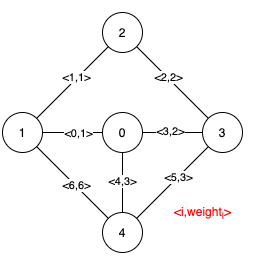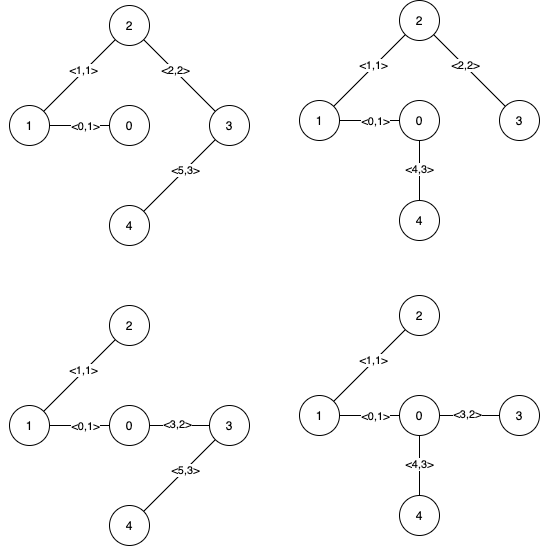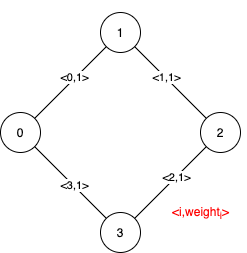# 找到最小生成树里的关键边和伪关键边

## 1489. 找到最小生成树里的关键边和伪关键边 (Hard)```输入：n = 5, edges = [[0,1,1],[1,2,1],[2,3,2],[0,3,2],[0,4,3],[3,4,3],[1,4,6]]``````输入：n = 4, edges = [[0,1,1],[1,2,1],[2,3,1],[0,3,1]]

```

• `2 <= n <= 100`
• `1 <= edges.length <= min(200, n * (n - 1) / 2)`
• `edges[i].length == 3`
• `0 <= fromi < toi < n`
• `1 <= weighti <= 1000`
• 所有 `(fromi, toi)` 数对都是互不相同的。

[深度优先搜索] [并查集]